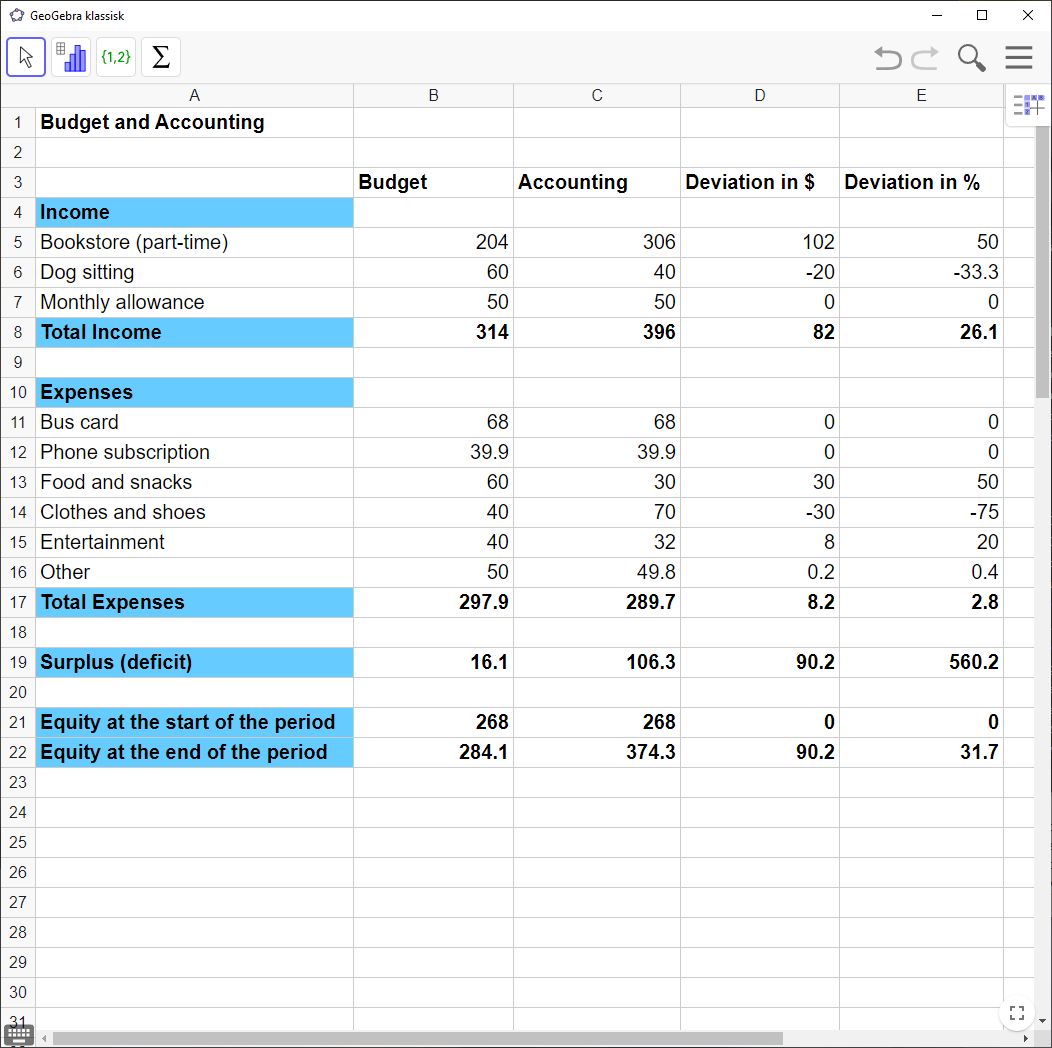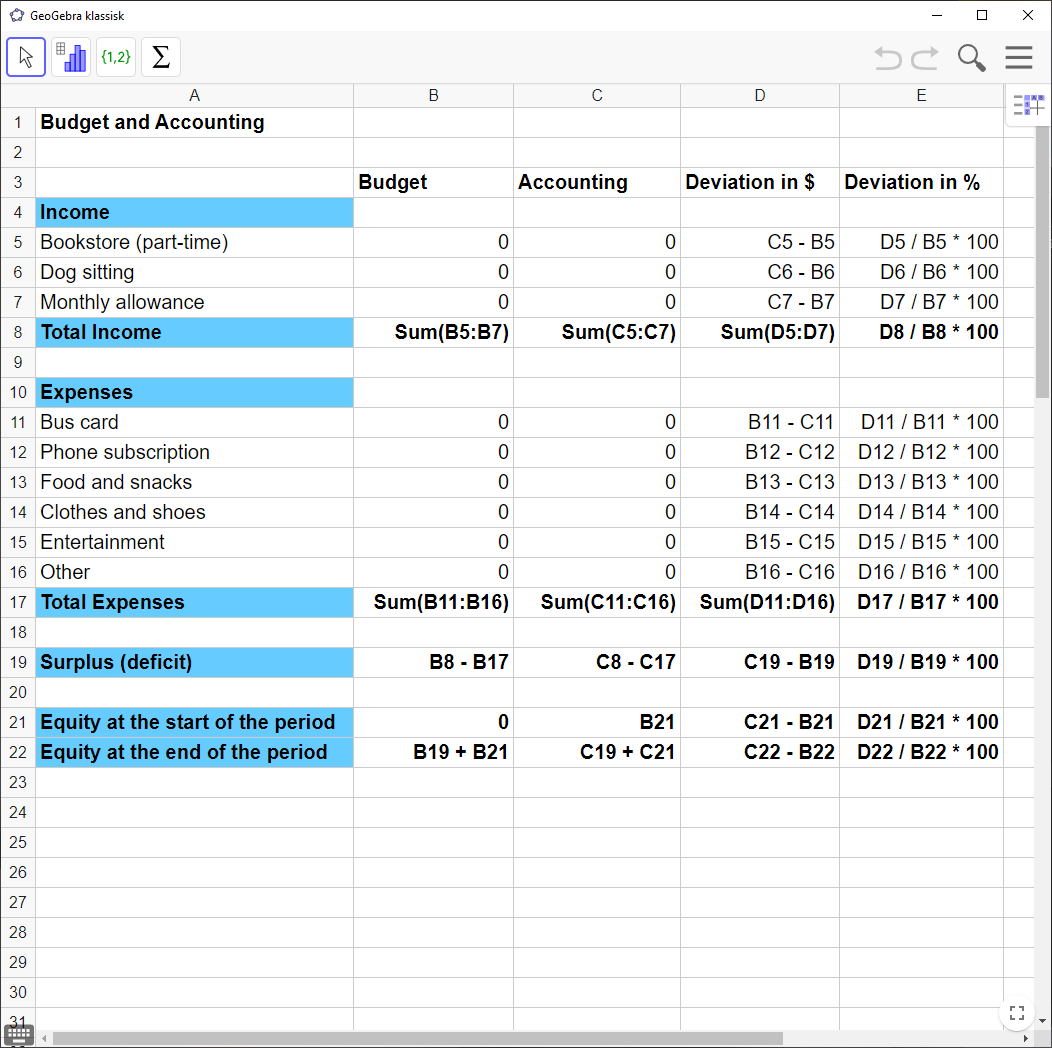# Spreadsheets for Budget and Accounting in GeoGebra

Here I show how to use `Spreadsheet` in `GeoGebra` to create and compare your budget and accounting. Sometimes it might be more convenient to use `Excel` .

Example 1

Erica Clementine is making an overview of all income and expenses she expects in a certain month. Her budget is given below.

 Income Amount Expenses Amount Bookstore (part-time) \$$240$ Bus card \$$68$ Walking dogs \$$60$ Phone subscription \$$39$ Pocket money \$$50$ Food and snacks \$$60$ Clothes and shoes \$$40$ Entertainment \$$40$ Other \$$50$

Towards the end of the month, she did her accounting and entered the actual income and expenses during the month. Erica has the following accounting figures:

 Income Amount Expenses Amount Bookstore (part-time) \$$300$ Bus card \$$68$ Dog sitting \$$40$ Phone subscription \$$39$ Monthly allowance \$$50$ Food and snacks \$$30$ Clothes and shoes \$$70$ Entertainment \$$32$ Other \$$49$

In addition, she already had \$$268$ in her bank account at the start of the month.

She compares her budget and accounting in `GeoGebra`. The final result looks like this:Follow the instructions below to learn how you can make your own budget and accounting, just like Erica’s above. Here is the template she used:Note! When you enter your own income and expenses, you’ll often get a spreadsheet that looks a bit different than the one in the template, unless you have the same income and expenses as Erica. But if you follow the instructions below, you’ll always get a correct spreadsheet.

`GeoGebra` Instruction 1

### Income

1.
Open `Spreadsheet` in`Menu`.
2.
In column `A`, enter the entries for the income in your budget.
3.
You start by entering “Income” as the column header. Below will be all the names of the various incomes. When all the entries for income have been listed under the header, finish by typing “Total Income” on the line below.
4.
In column `B`, type “Budget” as the header (preferably on a line higher than where you typed “Income”).
5.
Fill in the budget figures from the exercise so that they match the entries for income.
6.
In column `C`, type “Accounting” as the header, so that it has the same row number as “Budget”.
7.
Fill in the accounting figures from the exercise so that they match the income names.
8.
In column `D`, type “Deviation in \$” as the heading, so that it has the same row number as “Budget”.
9.
In column `E`, type “Deviation in %” as the heading, so that it gets the same row number as “Budget”.
10.
You will find “Total Income” by selecting all the cells with “Income” entries, and then using the command `Sum`. You must do this column by column for “Budget”, “Accounting” and “Deviation in \$”.

Look at Example 1 and the pictures below when working with the instructions. There you find one picture with the numbers and one picture with the formulas.

`GeoGebra` Instruction 2

### Expenses

1.
In column `A`, enter the names of all the expenses you have in your budget.
2.
Start by entering “Expenses” as the heading, and then list all the names of the various expenses below. When all the entries for expense are entered, finish by typing “Total Expenses” in the line below.
3.
In column `B`, enter the budget figures from the exercise so that they match the name of the expenses.
4.
In column `C`, enter the accounting figures from the exercise so that they match the names of the expenses.
5.
You can find “Total Expenses” by selecting all the cells with the values of “Expenses”, which are below each other, and pressing `Sum`. You must do this column by column for “Budget”, “Accounting” and “Deviation in \$” in order to have all the totals.

`GeoGebra` Instruction 3

### Deviationsin\$andDeviationsin%

1.
You can find “Deviation in \$” for “Income” by typing `=`. Then tap the cell that contains the value of “Income”, which is under “Accounting”. Then press the minus sign, and then click on the cell that contains the value of “Income”, which is under “Budget”. Press `Enter`.

= <the cell with the value of “Income” in “Accounting”> $-$ <the cell with the value of “Income” in “Budget”>

`Enter`

2.
You can find “Deviation in \$” for “Expenses” by typing `=`. Then press the cell that contains the value of “Expenses”, which is under “Budget”. Then press the minus sign, and then the cell that contains the value of “Expenses”, which is under “Accounting”. Press `Enter`.

= <the cell with the value of “Expenses” in “Budget”> $-$ <the cell with the value of “Expenses” in “Accounting”>

`Enter`

3.
You find “Deviation in %” for “Income” and “Expenses” by typing `=`. Then press the cell that contains the value of “Deviation in \$”. Then press the punctuation mark, and then the cell that contains the value of “Revenue” or “Expenses”, which is under “Budget”. Multiply this by 100 and press `Enter`. You need to do this in turn for all the different entries for income and expenses.

= <the cell with the value of “Deviation in \$”> / <the cell with the value of “Income” (“Expenses”) in “Budget”> * 100

`Enter`

`GeoGebra` Instruction 4

### SurplusandDeficitforBudgetandAccounting

1.
You’ll find “Surplus / Deficit” by clicking the cell on the right and typing `=` and clicking the cell containing the value of “Total Income”, then the minus sign. Then press the cell that contains the value of “Total Expenses”. Press `Enter`.
2.
You need to do this column by column for “Budget” and “Accounting”.

Note! Positive numbers mean surplus and negative numbers mean deficit.

`GeoGebra` Instruction 5

### SurplusandDeficitforDeviationsin\$

You will find “Surplus / Deficit” by clicking on the cell on the right and typing `=` and clicking on the cell that contains the value of “Total Income”. Then press the plus sign, then click on the value of `Total Expenses`. Press `Enter`.

### Surplus and Deficit for Deviations in %

You can find “Deviation in %” by typing `=`, and then clicking on the cell that contains the value of “Surplus / Deficit”, which is below “Deviation in \$”. Then press the division sign / and click on the cell that contains the value of “Surplus / Deficit”, which is under “Budget”. Press `Enter`.

`GeoGebra` Instruction 6

### MoneyattheBeginningandEndoftheMonth

1.
In column `A`, below your setup, type “Money at the Beginning of the Month” and “Money at the End of the Month”.
2.
“Money at the Beginning of the Month” is usually given in the exercise. Enter this directly in column `B`.
3.
You find “Money at the End of the Month” $=$ “Money at the Beginning of the Month” $+$ “Surplus / Deficit”, both of which are under “Accounting”. This is done by typing `=` and clicking on the cell that contains the value of “Money at the Beginning of the Month”, then pressing the plus sign, and then clicking on the cell that contains “Surplus / Deficit”, which is under “Accounting”. Press `Enter`. Explicitly,

= <the cell with the value of “Money at the Beginning of the Month”>

$+$ <the cell with the value of “Surplus / Deficit” in “Accounting”>

`Enter`

To get a better overview of the actual deviation between the budget and the accounting, you should find the deviation both in dollars and in percent.